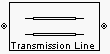# Transmission Line (Equivalent Baseband)

Model general transmission line

## Library

Transmission Lines sublibrary of the Physical library

•## Description

The Transmission Line block models the transmission line described in the block dialog box in terms of its physical parameters. The transmission line, which can be lossy or lossless, is treated as a two-port linear network.

The block enables you to model the transmission line as a stub or as a stubless line.

### Stubless Transmission Line

If you model the transmission line as a stubless line, the Transmission Line block first calculates the ABCD-parameters at each frequency contained in the modeling frequencies vector. It then uses the `abcd2s` function to convert the ABCD-parameters to S-parameters.

The block calculates the ABCD-parameters using the physical length of the transmission line, d, and the complex propagation constant, k, using the following equations:

`$\begin{array}{l}A=\frac{{e}^{kd}+{e}^{-kd}}{2}\\ B=\frac{{Z}_{0}*\left({e}^{kd}-{e}^{-kd}\right)}{2}\\ C=\frac{{e}^{kd}-{e}^{-kd}}{2*{Z}_{0}}\\ D=\frac{{e}^{kd}+{e}^{-kd}}{2}\end{array}$`

Z0 is the specified characteristic impedance. k is a vector whose elements correspond to the elements of the input vector `freq`. The block calculates k from the specified parameters as k = αa + , where αa is the attenuation coefficient and β is the wave number. The attenuation coefficient αa is related to the specified loss, α, by

`${\alpha }_{a}=-\mathrm{ln}\left({10}^{\alpha /20}\right)$`

The wave number β is related to the specified phase velocity, Vp, by

`$\beta =\frac{2\pi f}{{V}_{p}}$`

The phase velocity VP is also known as the wave propagation velocity.

### Shunt and Series Stubs

If you model the transmission line as a shunt or series stub, the Transmission Line block first calculates the ABCD-parameters at each frequency contained in the vector of modeling frequencies. It then uses the `abcd2s` function to convert the ABCD-parameters to S-parameters.

### Shunt ABCD-Parameters

When you set the Stub mode parameter in the mask dialog box to `Shunt`, the two-port network consists of a stub transmission line that you can terminate with either a short circuit or an open circuit as shown here.Zin is the input impedance of the shunt circuit. The ABCD-parameters for the shunt stub are calculated as

`$\begin{array}{c}A=1\\ B=0\\ C=1/{Z}_{in}\\ D=1\end{array}$`

### Series ABCD-Parameters

When you set the Stub mode parameter in the mask dialog box to `Series`, the two-port network consists of a series transmission line that you can terminate with either a short circuit or an open circuit as shown here.Zin is the input impedance of the series circuit. The ABCD-parameters for the series stub are calculated as

`$\begin{array}{c}A=1\\ B={Z}_{in}\\ C=0\\ D=1\end{array}$`

## Parameters

### Main Tab

Characteristic impedance (ohms)

Characteristic impedance of the transmission line. The value can be complex.

Phase velocity (m/s)

Propagation velocity of a uniform plane wave on the transmission line.

Loss (dB/m)

Reduction in strength of the signal as it travels over the transmission line. Must be positive.

Frequency (Hz)

Vector of modeling frequencies. The block performs the calculations listed in the Description section at each frequency you provide.

Transmission line length (m)

Physical length of the transmission line.

Stub mode

Type of stub. Choices are `Not a stub`, `Shunt`, or `Series`.

Termination of stub

Stub termination for stub modes `Shunt` and `Series`. Choices are `Open` or `Short`. This parameter becomes visible only when Stub mode is set to `Shunt` or `Series`.

### Visualization Tab

For information about plotting, see Create Plots.

## References

 Ludwig, Reinhold and Pavel Bretchko, RF Circuit Design: Theory and Applications, Prentice-Hall, 2000.